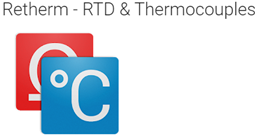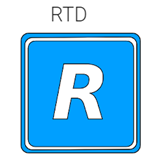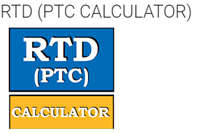# 5 – Best RTD converter apps for android and their features

1. Retherm-RTD & ThermocouplesThis app can convert resistance from RTD sensors to temperature. It can be used to convert Pt100, Pt500, and Pt1000 resistance to degree Celsius or Fahrenheit. Mostly the equations are done according to the European standard for the temperature range of -200 degree Celsius to + 850 degrees Celsius. This app can also be used to convert thermocouples voltages to degrees

2. PT100 converterThis converter can convert the RTD resistance reading to temperature and vice versa. It can do conversion between Celsius to Fahrenheit and upon each conversion, we can see the nearest values too. The PT converter will use certain equations to find out the required resistance and temperature and the same method is used to generate the OHM/ Temperature tables. This app will be very useful for industrial technicians

3. RTDThis app can be used to calculate the temperature from the resistance measurement. Outside air temperature measurement is crucial. With the help of this app, we can do proper airspeed measurements, and this app is really good for OAT measurements. This app can calculate the temperature measurements from any RTD sensor no matter what is the resistance value at zero degree Celsius PT/RTD100, PT/RTD500, PT/RTD1000 are all included.

4.RTD(PTC calculator)By using this app we can convert to PT100, PT500, PT1000 adding the temperature in degree Celsius and Fahrenheit. We can also select different materials such as platinum, copper, nickel, and iron or add the coefficient we need. This is the perfect app for instrument technicians